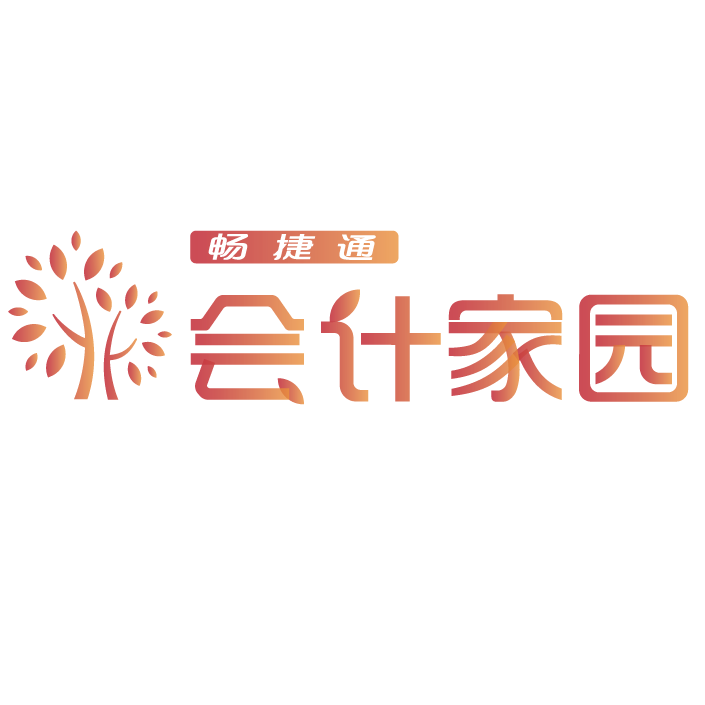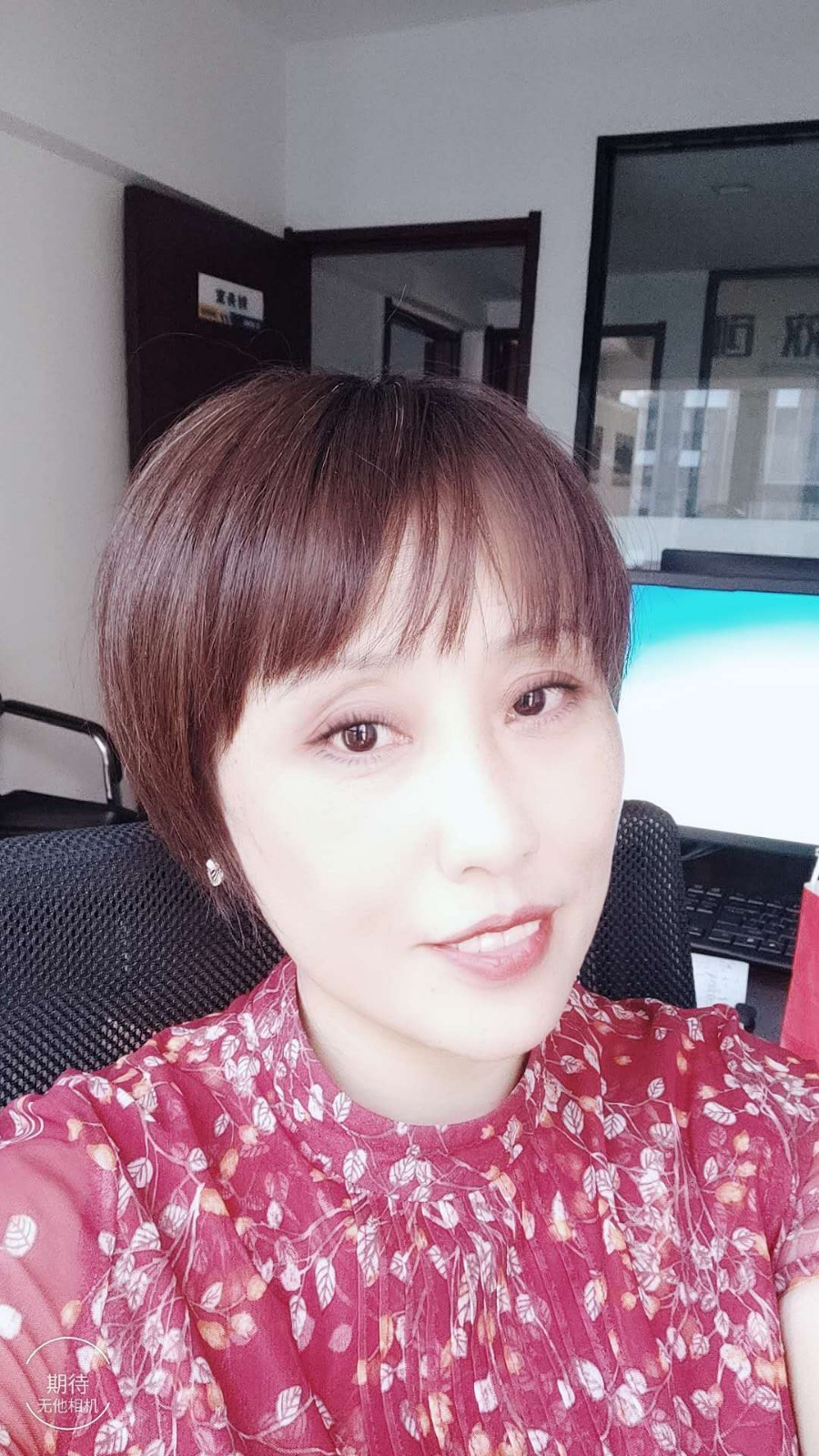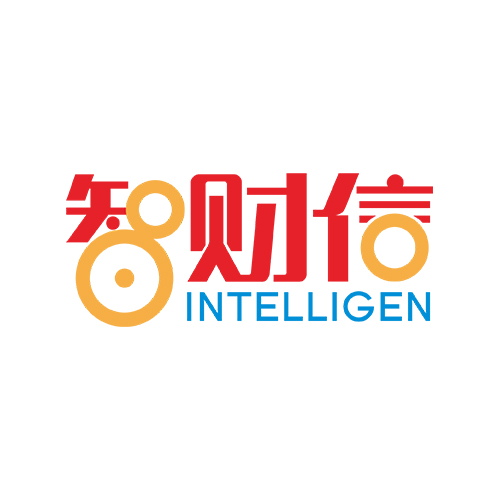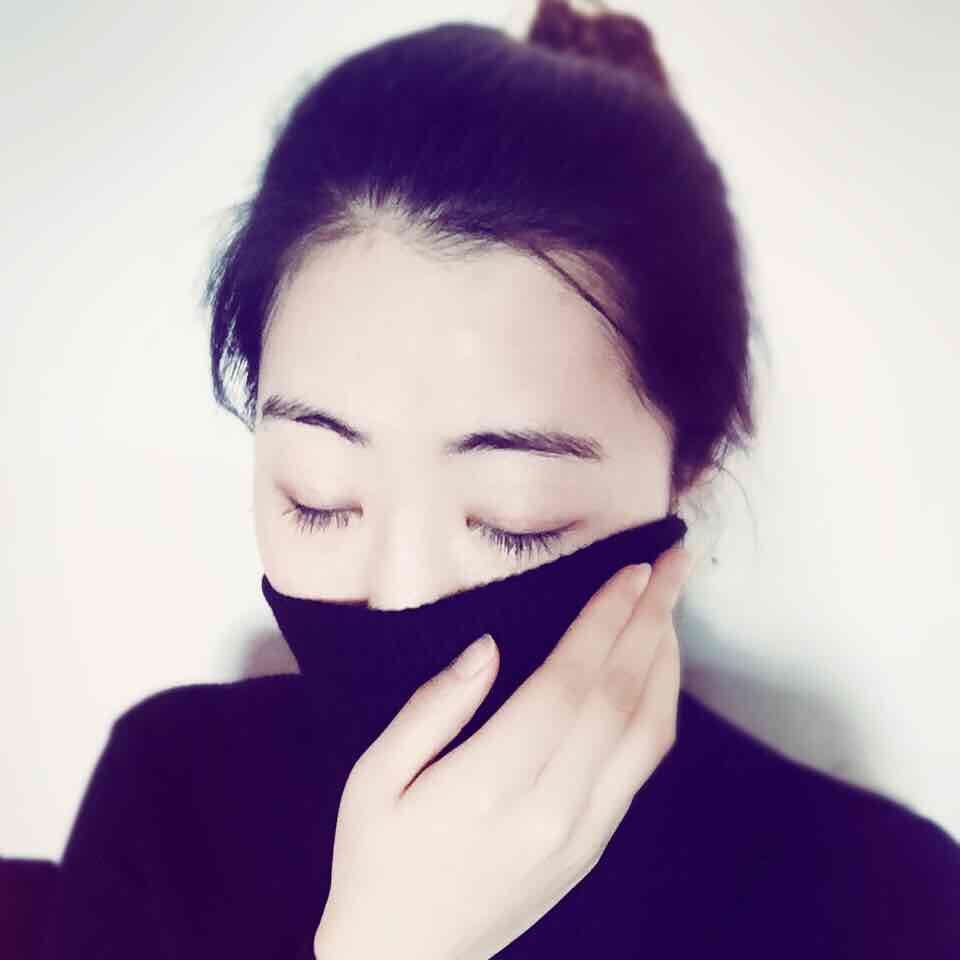### 2021中级会计 《财务管理》 考前冲刺试题及解析

`中级会计职称练习题，巩固所学知识点。`

1、下列关于成本管理的主要内容的说法中，错误的有( )。

A.成本预测是进行成本管理的第一步

B.成本决策对其他营运决策起着指导和约束作用

C.成本分析只是成本管理的某一阶段

D.成本考核的指标只能是财务指标

[答案]CD

[解析]成本分析贯穿于成本管理的全过程，选项C的说法错误。成本考核的指标可以是财务指标，也可以是非财务指标，选项D的说法错误。

2、在多品种条件下，能够影响综合边际贡献率大小的因素有( )。

A.企业固定成本总额

B.各种产品销售收入比重

C.各种产品的边际贡献率

D.全厂目标利润

[答案]BC

[解析]综合边际贡献率=∑各产品边际贡献÷∑各产品销售收入=∑(各产品边际贡献率×各产品销售收入比重)，选项B、C能够影响综合边际贡献率的大小。

3、某公司生产销售A、B、C三种产品，销售单价分别为20元、25元、10元;预计销售量分别为3000件、2000件、1500件;预计各产品的单位变动成本分别为12元、14元、8元;预计固定成本总额为245万元。按联合单位法(产品销量比为A：B：C=6：4：3)确定各产品的盈亏平衡点的销售量和盈亏平衡点的销售额，下列说法中正确的有( )。

A.联合单价为250元

B.联合单位销售量为500件

C.联合盈亏平衡点的业务量为2.5万件

D.A产品的盈亏平衡点的销售额为300万元

[答案]ABCD

[解析]联合单价=6×20+4×25+3×10=250(元);联合单位销售量=1500/3=500(件)，或3000/6=500(件)，或2000/4=500(件);联合单位变动成本=6×12+4×14+3×8=152(元)，联合单位边际贡献=250-152=98(元)，联合盈亏平衡点的业务量=245/98=2.5(万件)，A产品的盈亏平衡点的销售量=2.5×6=15(万件)，A产品的盈亏平衡点的销售额=15×20=300(万元)。

4、某公司生产销售A、B、C三种产品，销售单价分别为20元、24元、18元;预计销售量分别为1500件、2000件、1000件;预计各产品的单位变动成本分别为12元、14元、10元;预计固定成本总额为170万元。按分算法(按边际贡献的比重分配)确定各产品的盈亏平衡点的销售量和盈亏平衡点的销售额，下列说法中正确的有( )。

A.A产品的边际贡献比重为30%

B.分配给A产品的固定成本为51万元

C.A产品的盈亏平衡点的销售量为63750件

D.A产品的盈亏平衡点的销售额为127.5万元

[答案]ABCD

[解析]综合边际贡献=1500×(20-12)+2000×(24-14)+1000×(18-10)=40000(元)，A产品的边际贡献比重=1500×(20-12)/40000=30%，分配给A产品的固定成本=170×30%=51(万元)，A产品的盈亏平衡点的销售量=51/8=6.375(万件)=63750(件)，A产品的盈亏平衡点的销售额=6.375×20=127.5(万元)。

5、某企业只生产一种产品，单价为10元，单位变动成本为6元，固定成本5000元，销量1000件。欲实现目标利润2000元，可以采取的措施不包括( )。

A.单价提高到12元，其他条件不变

B.单位变动成本降低至3元，其他条件不变

C.固定成本降低至4000元，其他条件不变

D.销量增加至1500件，其他条件不变

[答案]ACD

[解析]利润=销售量×(单价-单位变动成本)-固定成本。设单价为P，则2000=(P-6)×1000-5000，可得P=13元，故选项A是正确答案;设单位变动成本为V，则2000=(10-V)×1000-5000，V=3元，故选项B不正确;设固定成本为F，则2000=(10-6)×1000-F，F=2000元，故选项C是正确答案;设销售量为Q，则2000=(10-6)×Q-5000，Q=1750件，故选项D是正确答案。

6、甲公司只生产销售一种产品——A产品，单价为50元/件，2019年生产与销售A产品10万件，全年发生变动成本240万元，固定成本130万元，单位变动成本为30元。

要求：

(1)计算A产品的边际贡献总额、盈亏平衡点的销售量;

(2)计算A产品的盈亏平衡作业率、安全边际。

[答案](1)A产品的边际贡献总额=销售收入-变动成本=50×10-240=260(万元)

A产品的盈亏平衡点的销售量=固定成本/(单价-单位变动成本)=130/(50-240/10)=5(万件)

(2)A产品的盈亏平衡作业率=盈亏平衡点销售量/正常经营销售量(实际销售量)=5/10=50%

A产品的安全边际额=正常销售额(实际销售额)-盈亏平衡点销售额=10×50-5×50=250(万元)

2[.问答题]乙公司是一家食品生产企业，生产A、B、C三种产品，销售单价分别为5元、7.5元、10元，预计销售量分别为90000件、70000件、40000件，预计各种产品的单位变动成本分别为3元、5元、6元，预计固定成本总额为420000元。

要求：

(1)计算各种产品的边际贡献率;

(2)按加权平均法进行产品组合盈亏平衡分析，计算各种产品的盈亏平衡点的销售额。

[答案](1)A产品的边际贡献率=(销售单价-单位变动成本)/销售单价=(5-3)/5=40%

B产品的边际贡献率=(销售单价-单位变动成本)/销售单价=(7.5-5)/7.5=33.33%

C产品的边际贡献率=(销售单价-单位变动成本)/销售单价=(10-6)/10=39.39%

(2)A产品的销售比重=90000/(90000+70000+40000)=45%

B产品的销售比重=70000/(90000+70000+40000)=35%

B产品的销售比重=40000/(90000+70000+40000)=20%

乙公司的综合边际贡献率=40%×45%+33.33%×35%+39.39%×20%=37.55%

乙公司的综合盈亏平衡点的销售额

=固定成本总额/综合边际贡献率

=420000/37.55%=1118508.66(元)

A产品盈亏平衡点的销售额=1118508.66×45%=503328.9(元)

B产品盈亏平衡点的销售额=1118508.66×35%=391478.03(元)

C产品盈亏平衡点的销售额=1118508.66×20%=223701.73(元)

## 写作榜 »

1.是我的海 1409 文章
2.老会计卓玛 1389 文章
3.璞之-会计家园 1057 文章
4.倪老师 533 文章
5.我是二娃 51 文章
6.尔玉 33 文章
7.程守峰【武汉智财信】13971518806 27 文章
8.谢晶晶 22 文章